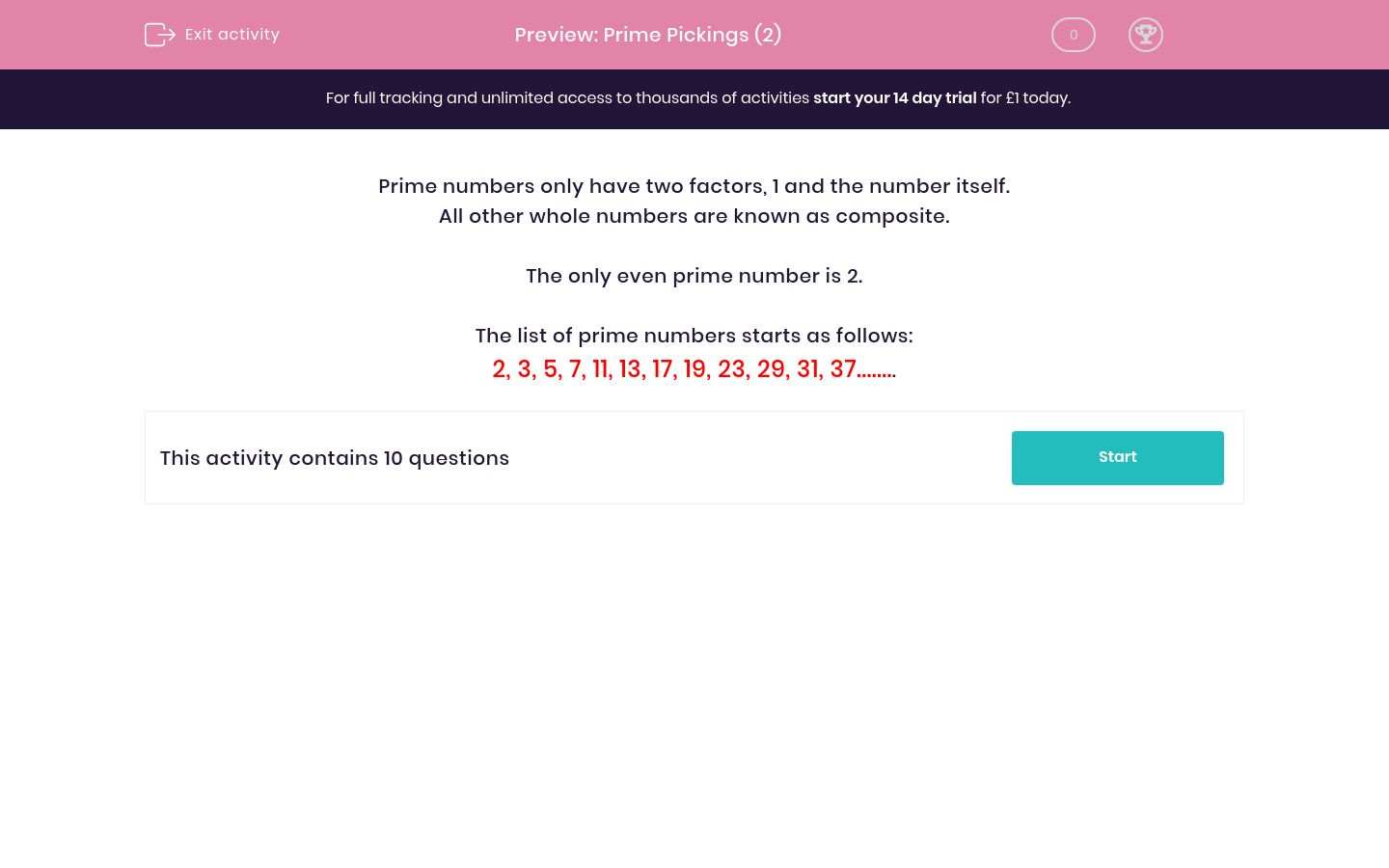# Prime Pickings (2)

In this worksheet, students select the numbers that are prime.Key stage:  KS 2

Curriculum topic:   Maths and Numerical Reasoning

Curriculum subtopic:   Factors and Multiples

Difficulty level:### QUESTION 1 of 10

Prime numbers only have two factors, 1 and the number itself.

All other whole numbers are known as composite.

The only even prime number is 2.

The list of prime numbers starts as follows:

2, 3, 5, 7, 11, 13, 17, 19, 23, 29, 31, 37........

Select all the numbers which are prime.

52

55

58

59

61

Select all the numbers which are prime.

65

63

87

97

107

Select all the numbers which are prime.

98

97

79

89

99

Select all the numbers which are prime.

71

81

91

101

111

Select all the numbers which are prime.

31

79

103

107

109

Select all the numbers which are prime.

105

108

111

113

115

Select all the numbers which are prime.

123

125

127

129

131

Select all the numbers which are prime.

129

139

149

159

169

Select all the numbers which are prime.

273

171

267

175

179

Select all the numbers which are prime.

135

735

147

157

191

• Question 1

Select all the numbers which are prime.

59
61
• Question 2

Select all the numbers which are prime.

97
107
• Question 3

Select all the numbers which are prime.

97
79
89
• Question 4

Select all the numbers which are prime.

71
101
• Question 5

Select all the numbers which are prime.

31
79
103
107
109
• Question 6

Select all the numbers which are prime.

113
• Question 7

Select all the numbers which are prime.

127
131
• Question 8

Select all the numbers which are prime.

139
149
• Question 9

Select all the numbers which are prime.

179
• Question 10

Select all the numbers which are prime.

157
191
---- OR ----